Computational & Technology Resources
an online resource for computational,
engineering & technology publications
Civil-Comp Proceedings
ISSN 1759-3433
CCP: 84
PROCEEDINGS OF THE FIFTH INTERNATIONAL CONFERENCE ON ENGINEERING COMPUTATIONAL TECHNOLOGY
Edited by: B.H.V. Topping, G. Montero and R. Montenegro
Paper 89

Inexact Constraint Preconditioners for Optimization Problems

L. Bergamaschi1, M. Venturin2 and G. Zilli1

1Department of Mathematical Methods and Models for Scientific Applications,
2Department of Pure and Applied Mathematics,

Full Bibliographic Reference for this paper
L. Bergamaschi, M. Venturin, G. Zilli, "Inexact Constraint Preconditioners for Optimization Problems", in B.H.V. Topping, G. Montero, R. Montenegro, (Editors), "Proceedings of the Fifth International Conference on Engineering Computational Technology", Civil-Comp Press, Stirlingshire, UK, Paper 89, 2006. doi:10.4203/ccp.84.89
Keywords: interior-point methods, iterative solvers, preconditioners, approximate Jacobian.

Summary
In this paper we are concerned with the solution of large scale minimization problems subject to equality constraints, via interior point methods with iterative solvers. When minimization problems subject to equality constraints are considered, each iteration of an interior point method requires the following linear system of equations to be solved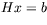where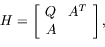(77)

where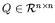is the Hessian of Lagrangian and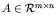is the Jacobian of constraints. The matrix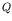arising in interior point applications has form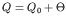, where diagonal scaling matrix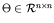with strictly positive elements (due to the barrier terms for primal variables). For linear programs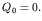In general we assume that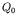is positive semidefinite (which impliespositive definite) and A has full row rank.

There has been growing interest in recent years in the use of iterative methods to solve the linear system (77). Although they involve significantly sparser factorizations than those used in direct approaches they still capture most of the numerical properties of the preconditioned system requiring less memory resources.

A variety of preconditioners have been proposed for such matrices. They have a common feature of constructing the approximation to (77) by simplifying its upper left block, namely by applying the preconditioner of the form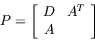(78)

where D is a sparse symmetric positive definite approximation to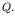This is a well studied problem with many possibilities [2,4]. Usual choices for matrix D are to keep it block-diagonal or diagonal. The latter situation has been studied for example in , where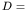diag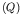.

The Hessian matrixcannot be approximated by anything simpler than a diagonal matrix. Consequently, the factorization of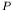(with a diagonal D) determines the least expensive constraint preconditioner among those preconditioners which "respect" constraints of the optimization problem. However, in certain situations such a preconditioner is still too expensive to compute.

In this paper we look for a further simplification of the preconditioner: we replace the Jacobian matrix A with a (sparse) approximation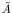. In the same direction, incomplete preconditioners are also used [3,1].

We propose to use the preconditioner of the following form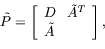(79)

where D is an approximation ofandis an approximation of A. We extend the analysis of [2,1] to this case and provide a complete characterisation of the spectrum of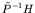. Moreover, we give some sufficient condition on the norm of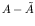to guarantee thathas maximum rank.

Dropping some of the elements in the Jacobian matrix A produces a significant reduction of the fill-in of the Cholesky factor of the preconditioner thus speeding-up the cost of a single iteration of the Krylov subspace method of choice. The spectral analysis of the preconditioned matrix reveals that a large number of eigenvalues are one or positive and bounded by those of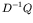. The distance of the remaining eigenvalues form unity is proven to be bounded in terms of the norm of the dropping matrix E. Some numerical results for a number of large quadratic problems demonstrate that the new approach is an attractive alternative for direct approach and for exact constraint preconditioners.

References
1
L. Bergamaschi, J. Gondzio, M. Venturin, and G. Zilli, Inexact constraint preconditioners for linear systems arising in interior point methods, Computational Opt. & Appls, (2006).
to appear. doi:10.1007/s10589-006-9001-0
2
L. Bergamaschi, J. Gondzio, and G. Zilli, Preconditioning indefinite systems in interior point methods for optimization, Computational Optimization and Applications, 28 (2004), pp. 149-171. doi:10.1023/B:COAP.0000026882.34332.1b
3
T. Coleman and A. Verma, A preconditioned conjugate gradient approach to linear equality constraned minimization, Computational Optimization and Applications, 20 (2001), pp. 61-72. doi:10.1023/A:1011271406353
4
C. Keller, N. I. M. Gould, and A. J. Wathen, Constraint preconditioning for indefinite linear systems, SIAM Journal on Matrix Analysis and Applications, 21 (2000), pp. 1300-1317. doi:10.1137/S0895479899351805

purchase the full-text of this paper (price £20)